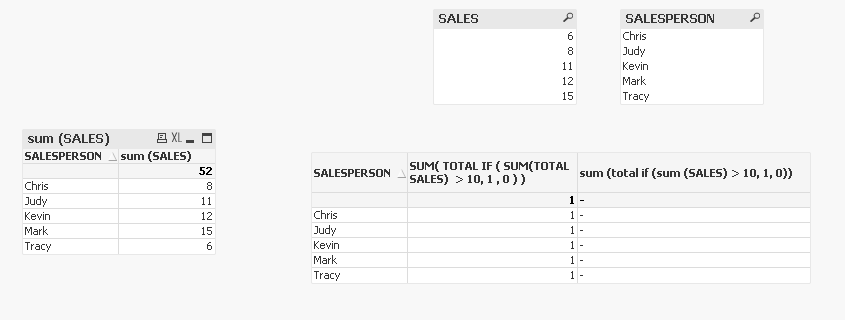# QlikView App Development

Discussion Board for collaboration related to QlikView App Development.Not applicable

## Sum (Total... with embedded IF?

Hello,

I'm trying to get the total of each column by using the sum(total.... command.  However I also have an embedded If statement and it's producing zero results.

So here is the formula I'm using is this:

sum (total if (sum SALES) > 10, 1, 0)

So I thought that this would produce a total of all rows that had sales greater than \$10, but I get zero results.

If I put in  just

if (sum SALES) > 10, 1, 0)

I get a result of 1 or 0 for each row (dimension SALESPERSON)

Any idea why when I add 'sum (total', that I get zero results / no sum ?

Thanks.

Tags (3)
1 Solution

Accepted SolutionsNot applicable

## Re: Sum (Total... with embedded IF?

hahah thanks... I came up with a similar solution

sum (TOTAL if (aggr (sum (SALES), SALESPERSON) > 10, 1, 0))

11 RepliesNot applicable

## Re: Sum (Total... with embedded IF?

sum (total if (sum SALES) > 10, 1, 0)  should be sum (total if (sum (SALES) > 10, 1, 0))

however that will just turned out to sum (total 1) or sum(total 0) which in turn will result in 1 or 0

is this a straight table ?  you can just write an expression like sum(sales) and put your dimensions accordingly and then check to add totals at the bottom and that will work automatically per column. if not please , explain with some sample data and the output you expectNot applicable

## Re: Sum (Total... with embedded IF?

yes it's a straight table (eventually to be a bar chart however with just the total), but I'm trying to display the same total (sum of all rows) in each row.

So lets say only 3 of the following salespeople below had sales over \$10, i would look like this

SALESPERSON        TOTAL SALES (ALL SALES PERSONS)

--------------                    --------------------------

Chris                                       3

Judy                                       3

Tracy                                      3

Kevin                                      3

Mark                                       3Not applicable

## Re: Sum (Total... with embedded IF?

dimension salesperson

expression sum(total sales)

doesn't work for you ?Not applicable

## Re: Sum (Total... with embedded IF?

sum (total sales) works just fine, but I'm trying to count total sales ONLY when the sale is over \$10.  So I have to have the If statement in there.....  I *thought* this would work, but it does not:

sum (total if (sum (SALES) > 10, 1, 0))

HighlightedNot applicable

## Re: Sum (Total... with embedded IF?

the SUM(TOTAL ) as you explained will be similar for all the rows, I am not sure what you are trying to accomplish but here is your expression:

SUM( TOTAL IF (

SUM(TOTAL SALES)  > 10, 1 , 0

)

)

or

SUM( TOTAL IF ( SUM(TOTAL SALES)  > 10, 1 , 0 ) )Not applicable

## Re: Sum (Total... with embedded IF?

Your formula produces a '1' for every row.  I'm trying to get a '3' for each row, which would denote that 3 salesperson exceeded \$10 in sales.

Below is both your formula (producing 1's for every row), and then my original formula, which is producing nothing.... do I need an AGGR function somehow?Not applicable

## Re: Sum (Total... with embedded IF?

Chris,

I have checked and here is the expression:

SUM(TOTAL AGGR(IF (SUM(SALES) > 10,1,0), SALESPERSON)

that should work.Not applicable

## Re: Sum (Total... with embedded IF?

hahah thanks... I came up with a similar solution

sum (TOTAL if (aggr (sum (SALES), SALESPERSON) > 10, 1, 0))

Honored Contributor III

## Re: Sum (Total... with embedded IF?

Dim: Salesperson

Expr: Count(TOTAL If(Aggr(Sum(Sales),Salesperson)>10,1))